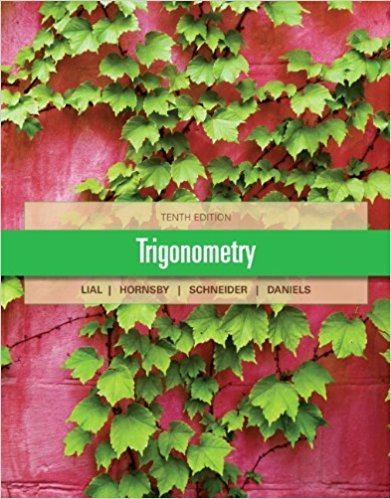×
×

# Solutions for Chapter 2.2: Trigonometric Functions of Non-Acute Angles## Full solutions for Trigonometry | 10th Edition

ISBN: 9780321671776Solutions for Chapter 2.2: Trigonometric Functions of Non-Acute Angles

Solutions for Chapter 2.2
4 5 0 276 Reviews
28
4
##### ISBN: 9780321671776

Since 90 problems in chapter 2.2: Trigonometric Functions of Non-Acute Angles have been answered, more than 35410 students have viewed full step-by-step solutions from this chapter. This expansive textbook survival guide covers the following chapters and their solutions. This textbook survival guide was created for the textbook: Trigonometry, edition: 10. Chapter 2.2: Trigonometric Functions of Non-Acute Angles includes 90 full step-by-step solutions. Trigonometry was written by and is associated to the ISBN: 9780321671776.

Key Math Terms and definitions covered in this textbook
• Block matrix.

A matrix can be partitioned into matrix blocks, by cuts between rows and/or between columns. Block multiplication ofAB is allowed if the block shapes permit.

• Circulant matrix C.

Constant diagonals wrap around as in cyclic shift S. Every circulant is Col + CIS + ... + Cn_lSn - l . Cx = convolution c * x. Eigenvectors in F.

• Diagonal matrix D.

dij = 0 if i #- j. Block-diagonal: zero outside square blocks Du.

• Dot product = Inner product x T y = XI Y 1 + ... + Xn Yn.

Complex dot product is x T Y . Perpendicular vectors have x T y = O. (AB)ij = (row i of A)T(column j of B).

• Ellipse (or ellipsoid) x T Ax = 1.

A must be positive definite; the axes of the ellipse are eigenvectors of A, with lengths 1/.JI. (For IIx II = 1 the vectors y = Ax lie on the ellipse IIA-1 yll2 = Y T(AAT)-1 Y = 1 displayed by eigshow; axis lengths ad

• Four Fundamental Subspaces C (A), N (A), C (AT), N (AT).

Use AT for complex A.

• Hankel matrix H.

Constant along each antidiagonal; hij depends on i + j.

• Hilbert matrix hilb(n).

Entries HU = 1/(i + j -1) = Jd X i- 1 xj-1dx. Positive definite but extremely small Amin and large condition number: H is ill-conditioned.

• Identity matrix I (or In).

Diagonal entries = 1, off-diagonal entries = 0.

• Iterative method.

A sequence of steps intended to approach the desired solution.

• Linearly dependent VI, ... , Vn.

A combination other than all Ci = 0 gives L Ci Vi = O.

• Nullspace N (A)

= All solutions to Ax = O. Dimension n - r = (# columns) - rank.

• Orthogonal matrix Q.

Square matrix with orthonormal columns, so QT = Q-l. Preserves length and angles, IIQxll = IIxll and (QX)T(Qy) = xTy. AlllAI = 1, with orthogonal eigenvectors. Examples: Rotation, reflection, permutation.

• Partial pivoting.

In each column, choose the largest available pivot to control roundoff; all multipliers have leij I < 1. See condition number.

• Reflection matrix (Householder) Q = I -2uuT.

Unit vector u is reflected to Qu = -u. All x intheplanemirroruTx = o have Qx = x. Notice QT = Q-1 = Q.

• Semidefinite matrix A.

(Positive) semidefinite: all x T Ax > 0, all A > 0; A = any RT R.

• Singular matrix A.

A square matrix that has no inverse: det(A) = o.

• Special solutions to As = O.

One free variable is Si = 1, other free variables = o.

• Subspace S of V.

Any vector space inside V, including V and Z = {zero vector only}.

• Unitary matrix UH = U T = U-I.

Orthonormal columns (complex analog of Q).

×